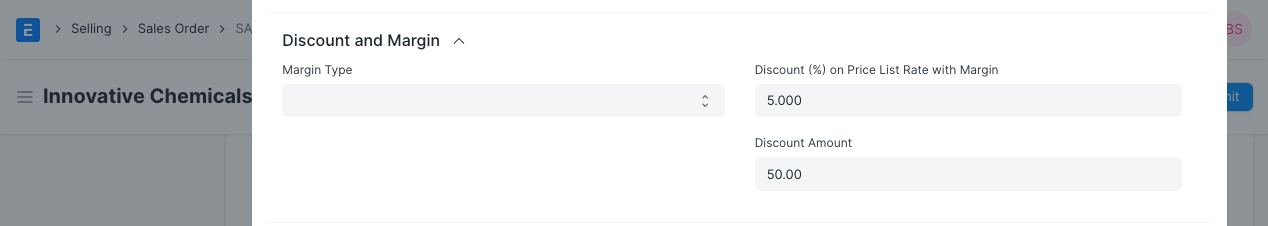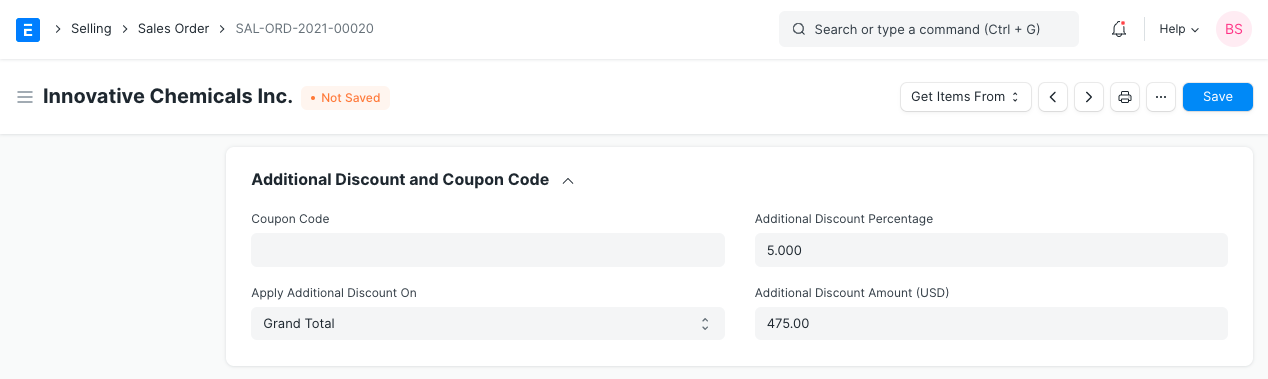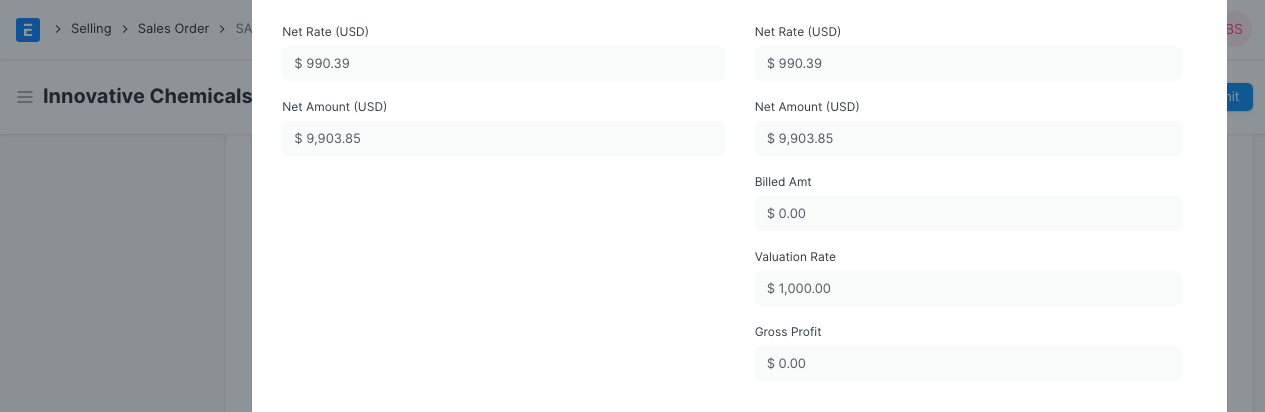# Applying a Discount

There are several ways to apply a Discount on an item in a sales transactions. This can be done in all sales and purchase transactions.

## 1. Discount on Price List Rate of an item

You can find the Discount field in the Item table of a transaction, click on the downward arrow at the righ-hand side of a row. A Discount can be applied as a percentage or a fixed amount related to the Price List Rate of the Item.The feature of Discount (%) is available in all sales and purchase transactions.

If you want to apply a discount (as a Percentage) regularly for certain quantities you'd rather use a "Pricing Rule". Read Pricing Rule documentation to learn more.

## 2. Discount on Net Total or Grand Total

In the "Additional Discount" section (of a "Sales Order" or "Sales Invoice" alike), you can apply a Discount as a fixed amount or a percentage on the total sum of the Sales.### 2.1 Discount on "Net Total"

If a Discount is applied on Net Total, then item's Net Rate and Net Amount is calculated as per the Discount Amount. Net Rate and Amount field will be visible only if Discount is applied using this feature.### 2.2 Discount on "Grand Total"

If a Discount is applied based on the Grand Total, then with item's Net Rate, Net Amount as well as taxes are also re-calculated as per Discount Amount.